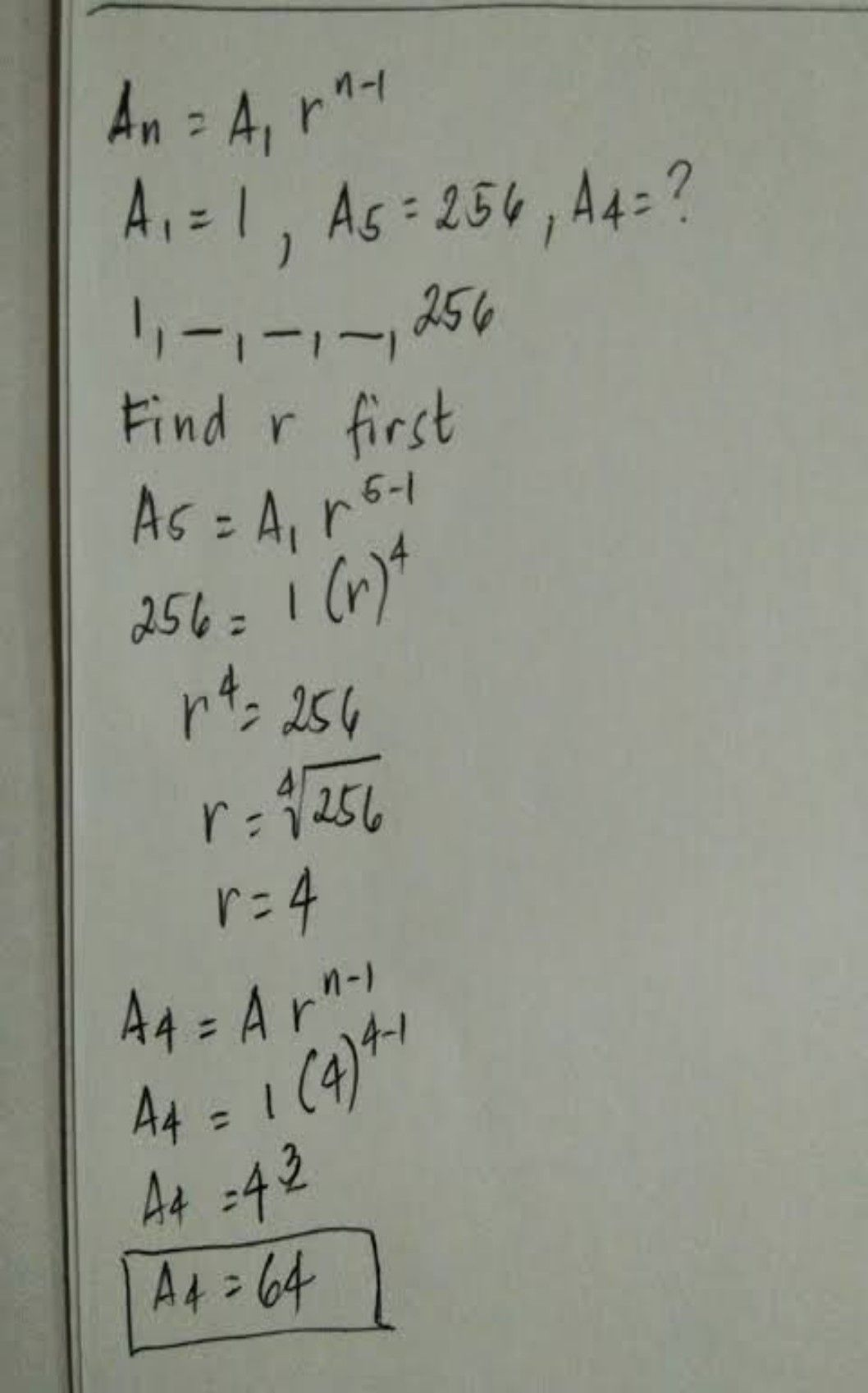Symbol
Problem$2$ tem $LE$ $1$ $p$ term $0$ 7th term $5$ If three geometric means are inserted between 1 and $256$ find the third geometric mean. $a$ $b4$ b. $32$ C. $16$ $0.$ $4$ 4
10th-13th grade
Algebra
Search count: 105
SolutionQanda teacher - vyshnaviStudent
Thank you maamQanda teacher - vyshnavi
please give me rating
go to evaluation and give rating
if u like provide some thank you gift coins